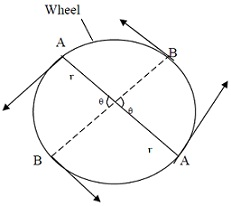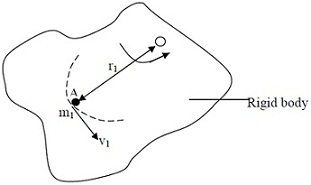#### Rigid Body Dynamics II, Physics tutorial

Torque:

It is time for us to ask what causes rotation. The analogies we have made between linear motion and rotational motion earlier in this course will be useful here. Recall that Newton's, second law describes the dynamics of linear motion whereby we have that a force causes linear motion given by an equation.

Here you will learn that rotational motion is caused by what we call a torque. You know that when we talk of a force, you intuitively think of a push or pull, so, in the case of torque. I would want you always to think of a twist. Know also that to increase the angular velocity of a rotating body, a torque of a couple must be applied. We see that torque is analogous to force.

F = Mdv/dt = ma

Where Torque,

Torque τ = dIω/dt = Idω/dt

It is often necessary to find the work done by a couple so that the energy exchange that takes place as a result of its action on a body can be known.

Consider a wheel. Let the radius of the wheel be r and two equal and opposite forces p act tangentially so that rotation occurs through angle Θ.

Now, Work done by each force = force x distanceHence Work done by each force = P x arc AB = pxrΘ

Hence Total work done by couple = PrΘ+ PrΘ = 2PrΘ

But torque or moment of couple = Px 2r = 2Pr

Therefore, work done by couple = torque x angle of rotation = τΘ

Angular Momentum:

Equivalent of liner momentum in rotational motion is angular momentum. Greater angular momentum of spinning objects like a top, greater its tendency to continue to spin.

Angular Momentum of the rotating body is proportional to its mass and to how quickly it is turning. Additionally angular momentum depends on how mass is distributed relative to axis of rotation: the further away mass is situated from axis of rotation, greater the angular momentum. The flat disk like the record turntable has more angular momentum than the tall cylinder of same mass and velocity of rotation.

Like linear momentum, angular momentum is vector quantity, and conservation implies that direction of spin axis tends to remain unchanged. For this cause spinning top remains upright while the stationary one falls over immediately.Let us consider the rigid body which is rotating about the axis O with the angular velocity ω at some moment of time. Let A be the particle of this body a distance r, from O axis of rotation. If particle has linear velocity V, as given in diagram then linear momentum of A is m1v1 = m1ωr1 ( since V1 = ωr1).

The angular momentum L of AO about O is then defined as the moment of momentum about O.

Hence,

Angular momentum L of A = r1 x m1ωr1

= ωm1r12

Therefore Total angular momentum = Σωmr2 of a rigid body

= ωΣmr2

Therefore L = 1ω

Where we identify 1 as moment of inertia of body about O. It is therefore obvious that angular momentum is analogue of linear momentum (mv) where 1 is equal to mass m and ω replaces velocity V.

We can then define Newton's second law of rotational dynamics as follows.

The body rotates when it is acted on by the couple.

Therefore τ = 1α

Where τ is the torque of moment of couple causing rotational acceleration α.

In terms of momentum we have that Torque = rate of change of angular momentum

i.e.

τ = Idω/dt = dl/dt

This is analogous to force that is the rate of change of linear momentum

F = mdv/dt

Conservation of Angular Momentum and Its Applications:

Angular momentum is the vector which points in same direction as w. For uniform rotational motion about the axis, angular momentum doesn't change in either magnitude or direction. Just as in case for linear momentum, angular momentum is independent of time for the system on which there is no torque because of external forces. It is possible that external torque is zero even when external force is not zero. This will rely on where external force is acted and on its direction. Likewise, net torque could exist when the net force is zero. When net torque is zero, the angular momentum is independent of time and is conserved. For rigid bodies, rotational inertia is constant, and conservation of angular momentum signifies that angular velocity is constant in time. When rotational inertia can differ because system considered can differ its shape, then conservation of angular momentum becomes extremely significant and helpful principle. Therefore principle of angular momentum defines that:

Total angular momentum of the system remains constant given no external torque applies on system rigid or otherwise. Mathematically we have that,

dL/dt = 0

Ice skaters, acrobats, ballet dancers, and divers use principle of conservation of angular momentum. For instance, diver leaves high diving board with outstretched arms and legs and some initial angular velocity about his centre of gravity. The angular momentum Iω remains constant as no external torques apply on him. To make the somersault he should increase his angular velocity. He does this by pulling in his legs and arms so that I decreases and ω thus increases. By extending arms and legs again, his angular velocity falls to the original value. Likewise the skater can whirl faster on ice by folding her arms.

Principle of conservation of angular momentum is helpful for handling with large rotating bodies like the earth, and small, spinning particles like electrons, protons, neutrons. Earth is an object that rotates about the axis passing through its geographic north and south poles with the period of 1 day. If it is hit by meteorites, then as action and reaction are equal, no external couple applies on earth and meteorites. Their total angular momentum is therefore conserved.

Neglecting angular momentum of meteorites about earth's axis before collision compared with that of earth. Then, Angular momentum of earth and meteorites after collision = angular momentum of earth before collision. As effective mass of earth has increased after collision moment of inertia has also increased. Therefore, earth will slow up slightly. Likewise, when the mass of object is dropped lightly on to the turntable rotating freely at the steady speed, conservation of angular momentum leads to the reduction in speed of turntable.

Top and Gyroscope:

The symmetrical body rotating about the axis, one point of which is fixed is known as a top. If fixed point is at centre of gravity, body is known as a gyroscope. The axis of rotation of top or gyroscope can itself rotate about fixed point so direction of the angular momentum vector can change.

Top (as the fixed point is not at centre of mass) is spinning about its axis of symmetry and if axis is at first set in motion in specific direction, with proper angular velocity, system carries on to rotate uniformly about pivot at O. Spin axis remains horizontal.

Angular momentum of the top would equal product of its moment of inertia about axis and the angular velocity about axis would point along it. If It's axis were set in space. But as the axis itself also rotates angular momentum no longer lies on axis. Though, if angular velocity of the axis itself is little compared to angular velocity about the axis, then component of angular momentum arising from former effect is small and can be neglected. Angular momentum vector L, about fixed point O, can then be drawn along the axis as shown and as the top rotates about O, its angular momentum vector rotates with it.

Upward force P at the pivot has no moment about O. Resultant external moment is that because of the weight w; its magnitude is

τ = ωR

Direction of τ is perpendicular to axis as shown. In a time Δt, this torque produces a change ΔL in the angular momentum, having the same direction as τ and given by

ΔL = τΔt

The angular momentum L + ΔL, after a time ΔL is perpendicular to L, new angular momentum vector has same magnitude as old but a different direction. As angular momentum vector lies along gyroscope axis, axis turns also, rotating in the horizontal plane about point O. This motion of axis of rotation is known as precession.

Tutorsglobe: A way to secure high grade in your curriculum (Online Tutoring)

Expand your confidence, grow study skills and improve your grades.

Since 2009, Tutorsglobe has proactively helped millions of students to get better grades in school, college or university and score well in competitive tests with live, one-on-one online tutoring.

Using an advanced developed tutoring system providing little or no wait time, the students are connected on-demand with a tutor at www.tutorsglobe.com. Students work one-on-one, in real-time with a tutor, communicating and studying using a virtual whiteboard technology.  Scientific and mathematical notation, symbols, geometric figures, graphing and freehand drawing can be rendered quickly and easily in the advanced whiteboard.

Free to know our price and packages for online physics tutoring. Chat with us or submit request at info@tutorsglobe.com